# Math in Focus Grade 5 Chapter 3 Practice 4 Answer Key Expressing Fractions, Division Expressions, and Mixed Numbers as Decimals

Go through the Math in Focus Grade 5 Workbook Answer Key Chapter 3 Practice 4 Expressing Fractions, Division Expressions, and Mixed Numbers as Decimals to finish your assignments.

## Math in Focus Grade 5 Chapter 3 Practice 4 Answer Key Expressing Fractions, Division Expressions, and Mixed Numbers as Decimals

Write each fraction as a decimal.

Example

$$\frac{3}{5}$$ = $$\frac{3}{5}$$
= 0.6

Question 1.
$$\frac{13}{20}$$ = ____
= _____
$$\frac{13}{20}$$ = $$\frac{13}{20}$$
= 0.65
Explanation:
Written the fraction as a decimal.

Question 2.
$$\frac{19}{25}$$ = ____
= _____
$$\frac{19}{25}$$ =$$\frac{19}{25}$$
= 0.76
Explanation:
Written the fraction as a decimal.

Question 3.
$$\frac{47}{50}$$ = ____
= _____
$$\frac{47}{50}$$ = $$\frac{47}{50}$$
= 0.94
Explanation:
Written the fraction as a decimal.

Express each division expression as a mixed number in simplest form and as a decimal.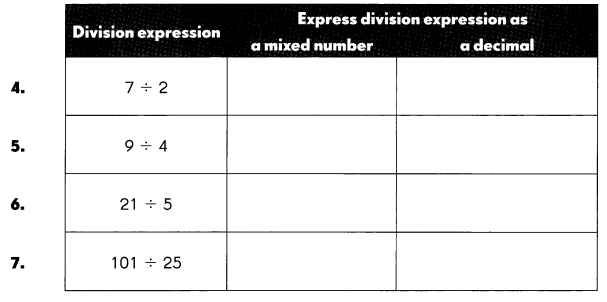Explanation: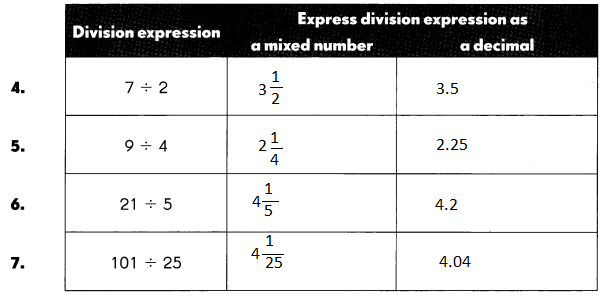Question 4.
7 ÷ 2
7 ÷ 2 = $$\frac{6}{2}$$ + $$\frac{1}{2}$$
= 3 + $$\frac{1}{2}$$
= 3 + 0.5
= 3.5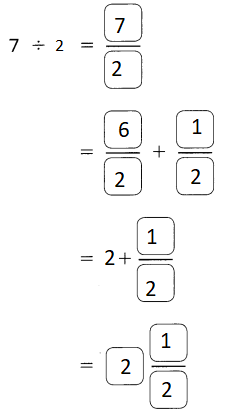Explanation:
Converted division expression into mixed fraction and decimal

Question 5.
9 ÷ 4
$$\frac{8}{4}$$ + $$\frac{1}{4}$$
= 2 + $$\frac{1}{4}$$
= 2 + 0.25
= 2.25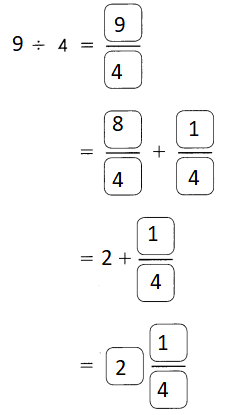Explanation:
Converted division expression into mixed fraction and decimal

Question 6.
21 ÷ 5
$$\frac{20}{5}$$ + $$\frac{1}{5}$$
= 4 + $$\frac{1}{5}$$
= 4 + 0.2
= 4.2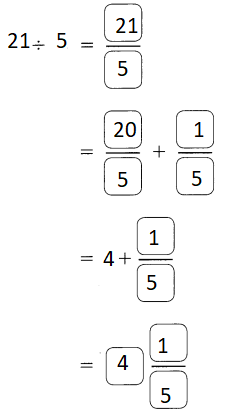Explanation:
Converted division expression into mixed fraction and decimal

Question 7.
101 ÷ 25
$$\frac{100}{25}$$ + $$\frac{1}{25}$$
= 1 + $$\frac{1}{25}$$
= 4 + 0.04
= 4.04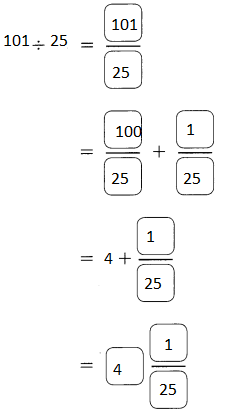Explanation:
Converted division expression into mixed fraction and decimal

Express each improper fraction as a decimal.

Example
$$\frac{3}{2}$$ = $$\frac{2}{2}$$ + $$\frac{1}{2}$$
= 1 + $$\frac{1}{2}$$
= 1 + 0.5
= 1.5
Explanation:
Converted each improper fraction into decimal

Question 8.
$$\frac{22}{5}$$
$$\frac{20}{5}$$ + $$\frac{2}{5}$$
= 4 + $$\frac{2}{5}$$
= 4 + 0.4
= 4.4
Explanation:
Converted each improper fraction into decimal

Question 9.
$$\frac{47}{20}$$
$$\frac{40}{20}$$ + $$\frac{7}{20}$$
= 2 + $$\frac{7}{20}$$
= 2 + 0.35
= 2.35
Explanation:
Converted each improper fraction into decimal

Question 10.
$$\frac{32}{25}$$
$$\frac{25}{25}$$ + $$\frac{7}{25}$$
= 1 + $$\frac{7}{25}$$
= 1 + 0.28
= 1.28
Explanation:
Converted each improper fraction into decimal

Question 11.
A coil of rope 603 feet long is cut into 25 equal pieces. What is the length of each piece? Express your answer as a mixed number and as a decimal.
24$$\frac{3}{25}$$ in mixed fraction.
603 ÷  25 = $$\frac{600}{25}$$ + $$\frac{3}{25}$$
= 24 + $$\frac{3}{25}$$
= 24$$\frac{3}{25}$$